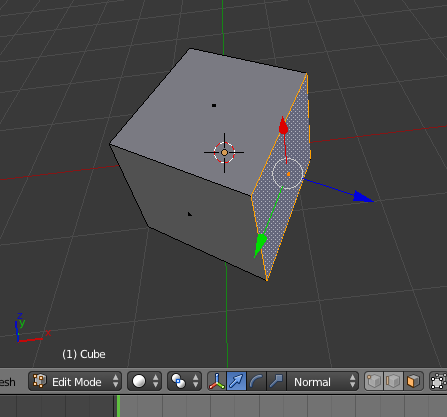# I want to get a matrix of Normal axix of selected face.

I want to get a matrix_world.## Is it possible?

``````    f_selected = obj.data.polygons
``````

## z = Vector((0,0,1)) angle = f_selected.normal.angle(z) axis = z.cross(f_selected.normal) mat_world_rot = Matrix.Rotation(angle, 4, axis)

How to get a matrix_world of normal axis of selected face.

Please answer to me.
Thank you.

well, that’s already the formula, just multiply with matrix_world of the object. Although there’s no real definition of a matrix for a vector

``````import bpy
from mathutils import Vector, Matrix

ob = bpy.context.object

if ob.type != 'MESH':
raise TypeError

me = ob.data
ob.update_from_editmode()

if len(me.polygons) &lt; 1:
raise ValueError

polys = me.polygons
poly_active = polys[polys.active]

z = Vector((0,0,1))
angle = poly_active.normal.angle(z)
axis = z.cross(poly_active.normal)
mat = Matrix.Rotation(angle, 4, axis)
mat_world = ob.matrix_world * mat

print(mat_world)

``````
2 Likes

Thank you your reply.